# RSI indicator - page 14340759

Nino Gannaban:
Hello anyone know what indicator this is? And if this is available

Why posting that in a RSI thread ?

And an other time please post a smaller image, I am still able to see it.181602

Alain Verleyen:

Why posting that in a RSI thread ?

And an other time please post a smaller image, I am still able to see it.

He already got an answer in PM that those are MACDs but obviously he did not like the answer :)40759

He already got an answer in PM that those are MACDs but obviously he did not like the answer :)
Ah he want it to be an RSI, got it :-D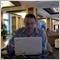Moderator
116833Moderator
116833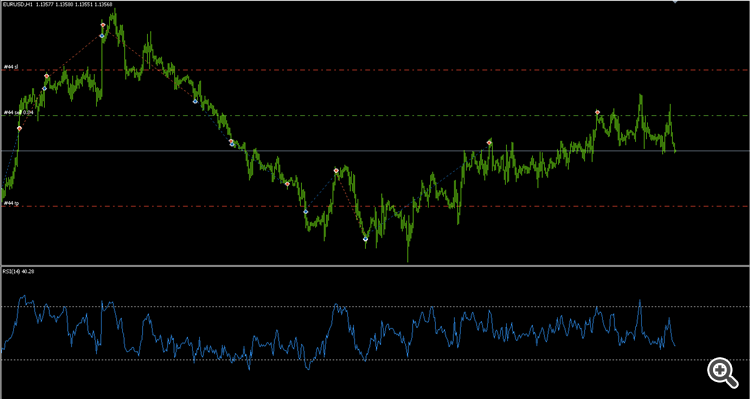Classic trading strategy based on RSI levels. Buys when RSI is oversold and sells when RSI is overbought.
This code can be used in both MetaTrader5 and MetaTrader4.  Just change the file extension from .mq5 to .mq4 and compile the file again.Moderator
116833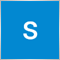29

Hello can anyone give me a rsi indicator where in it send push notifications when price crosses 75 and 25?Moderator
116833

Advisor works only on the new bar. The signal to open a position BUY: rsi on bar # 1> 'RSI Level UP'. Signal to open a SELL: rsi position on bar # 1 <'RSI Level DOWN'.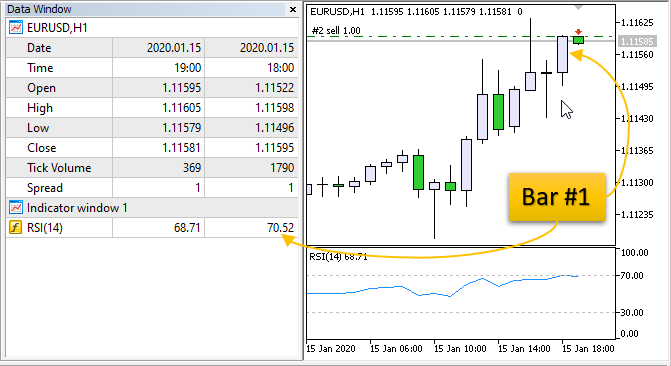```//+------------------------------------------------------------------+
//+------------------------------------------------------------------+
#property version   "1.005"
//---
//--- input parameters
input int                  Inp_RSI_ma_period       = 14;          // RSI: averaging period
input ENUM_APPLIED_PRICE   Inp_RSI_applied_price   = PRICE_CLOSE; // RSI: type of price
input double               Inp_RSI_Level_UP        = 70;          // RSI Level UP
input double               Inp_RSI_Level_DOWN      = 30;          // RSI Level DOWN
//---
int      handle_iRSI;                           // variable for storing the handle of the iRSI indicator
datetime m_prev_bars                = 0;        // "0" -> D'1970.01.01 00:00';
//+------------------------------------------------------------------+
//| Expert initialization function                                   |
//+------------------------------------------------------------------+
int OnInit()
{
//--- create handle of the indicator iRSI
handle_iRSI=iRSI(Symbol(),Period(),Inp_RSI_ma_period,Inp_RSI_applied_price);
//--- if the handle is not created
if(handle_iRSI==INVALID_HANDLE)
{
//--- tell about the failure and output the error code
PrintFormat("Failed to create handle of the iRSI indicator for the symbol %s/%s, error code %d",
Symbol(),
EnumToString(Period()),
GetLastError());
//--- the indicator is stopped early
return(INIT_FAILED);
}
//---
return(INIT_SUCCEEDED);
}
//+------------------------------------------------------------------+
//| Expert deinitialization function                                 |
//+------------------------------------------------------------------+
void OnDeinit(const int reason)
{
//---

}
//+------------------------------------------------------------------+
//| Expert tick function                                             |
//+------------------------------------------------------------------+
void OnTick()
{
//--- we work only at the time of the birth of new bar
datetime time_0=iTime(Symbol(),Period(),0);
if(time_0==m_prev_bars)
return;
m_prev_bars=time_0;
//---
double rsi_1=iRSIGet(1);
if(rsi_1==EMPTY_VALUE)
return;
//---
if(rsi_1>Inp_RSI_Level_UP)
else if(rsi_1<Inp_RSI_Level_DOWN)
}
//+------------------------------------------------------------------+
//+------------------------------------------------------------------+
{
//---
int d=0;
}
//+------------------------------------------------------------------+
//| Get value of buffers for the iRSI                                |
//+------------------------------------------------------------------+
double iRSIGet(const int index)
{
double RSI;
//--- reset error code
ResetLastError();
//--- fill a part of the iRSI array with values from the indicator buffer that has 0 index
if(CopyBuffer(handle_iRSI,0,index,1,RSI)<0)
{
//--- if the copying fails, tell the error code
PrintFormat("Failed to copy data from the iRSI indicator, error code %d",GetLastError());
//--- quit with zero result - it means that the indicator is considered as not calculated
return(EMPTY_VALUE);
}
return(RSI);
}
//+------------------------------------------------------------------+
```Moderator
116833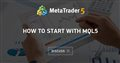• 2020.03.12
• www.mql5.com
This thread discusses MQL5 code examples. There will be examples of how to get data from indicators, how to program advisors...Moderator
116833

RSI with BB - indicator for MetaTrader 5

The indicator consists in an RSI and Bollinger bands calculated on it, it also plots arrows when RSI value was out of Bands and came again in Bands.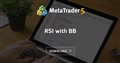RSI with BB
• www.mql5.com
The indicator consists in an RSI and Bollinger bands calculated on it, it also plots arrows when RSI value was out of Bands and came again in Bands.